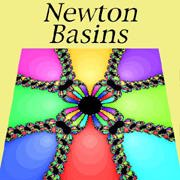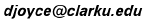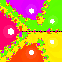# Newton Basins

### Some examples

David E. JoyceClark University
August, 1994

### A computer program to find Newton basins

It's pretty easy to write a program that colors Newton basins. Just decide how much of the complex plane to draw, and for each pixel in the image, iterate Newton's method on the corresponding complex number and see what happens. If some iterate gets close enough to a root, then you know which basin it belongs in. If after some number of times it hasn't gotten close to a root, then give up and go on to the next pixel. You can tell the computer what the maximum number of iterations it should consider. (If the maximum is too small, there will be a lot of uncolored pixels, and if it's too big it will take the computer too long a time to create the image.)

## The examples

Click on the thumbnail pictures to get larger images.The polynomial z4 - 1 has roots at 1, i, -1, and -i. White dots are placed around the roots.The polynomial z3 - 1 has the three roots of unity as its roots. The three roots are 1 (of course), -0.5 + 0.86603 i, and -0.5 - 0.86603 i. This and the last example are specific cases of the n-th roots of unity. They are the roots of the polynomial zn - 1. The n roots are equally spaced around the unit circle (that is, the circle whose equation is x2 + y2 = 1), so their coordinates can easily be given in terms of cosines and sines. The angles for these n points as measured counterclockwise from the positive x-axis are 0°, 360°/n, 360°·2/n, ..., 360° (n-1)/n, the k-th of these angles being 360° k/n. Therefore, the k-th root is cos (360° k/n) + i sin (360° k/n). In particular, the 0-th root is 1 itself.Even when the roots are all real, the Newton basins in the complex plane are interesting. In this example, the polynomial is z3 - z, and its roots are -1, 0, and 1.Here's an example where all the roots are complex but come in pairs. The six roots are ±(1+3i), ±(5+i), and ±(3-2i). The sixth degree polynomial is (z2 - (1+3i)2)(z2 - (5+i)2)(z2 - (3-2i)2).The polynomial (z4 - 1)(z4 + 16), that is, z8 + 15z4 - 16, has eight roots: ±1, ±i, ±(1+i), and ±(1-i). The roots are at the corners and the midpoints of the sides of the displayed square.This polynomial (z4 - 1)(z2 - (1+i)2) has six roots: ±1, ±i, and ±(1+i). The lighter colors indicate fewer iterations of Newton's method before the approximations are close to the roots.The polynomial (z2 + 4z + 1)(z2 +4)(z - 2), that is, z5 + 2z4 - 3z3 + 6z2 - 28 z - 8, has five roots: 2+i, 2-i, 2i, -2i, and -2.The polynomial (z4 - 1)(z4 - 16), that is, z8 - 17z4 + 16, has eight roots: ±1, ±i, ±2, and ±2i.Looking at the examples so far you'll get the impression that Newton's method almost always works. But sometimes that isn't so. Here's an example of a cubic polynomial for which lots of initial guesses never get close to any solution. Those initial guesses are colored black.The cubic polynomial for this image has two of the same roots as the last polynomial, but the third is slightly moved. The black area is a much more interesting shape.

Up to the Introduction
Back to Newton's method
On to the Generation form: create your own images!

The address of this file is http://aleph0.clarku.edu/~djoyce/newton/newton.html